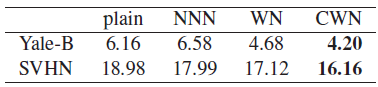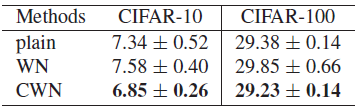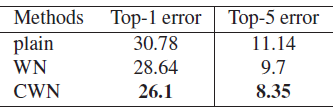# Review — CWN: Centered-Weight Normalization in Accelerating Training of Deep Neural Networks

## CWN, Re-parameterize Weight With Zero-Mean and Unit-Norm, Outperforms WN

Centered-Weight Normalization in Accelerating Training of Deep Neural Networks, CWN, by Beihang University, and The University of Sydney,
2017 ICCV, Over 50 Citations (Sik-Ho Tsang @ Medium)
Image Classification, Batch Normalization, BN, Weight Normalization, WN

• This paper proposes to reparameterize the input weight of each neuron in deep neural networks by normalizing it with zero-mean and unit-norm. That’s why it is called Centered-Weight Normalization (CWN).
• After normalization, it is followed by a learnable scalar parameter to further adjust the norm of the weight.

# Outline

1. Weight Normalization (Weight Norm, WN) Brief Review
2. Centered-Weight Normalization (CWN)
3. Experimental Results

# 1. Weight Normalization (Weight Norm, WN) Brief Review

• (Please skip this section if you know Weight Norm, WN, well.)
• Basically, the original input weight vectors v are normalized by its Euclidean norm of v,||v||, then a scalar parameter g is re-weight v again to obtain w:

By decoupling the norm of the weight vector g from the direction of the weight vector (v/||v||), the convergence of stochastic gradient descent optimization is speed up.

# 2. Centered-Weight Normalization (CWN)

• In CWN, the original input weight vector v is first re-parameterized and make sure that it has the following properties.
• Zero-mean, with 1 is a column vector of all ones:
• Unit-norm, with ||w|| denotes the Euclidean norm of w:
• To achieve this goal, below equation is used:
• where d is the dimension of the input weight.

With centered weight normalization (CWN), we center and scale the input parameter v to ensure that the input weight w has the desired zero-mean and unit-norm properties.

• While these constraints provide regularization, they also may reduce the representation capacity of the networks. To address it, a learnable scalar parameter g is simply introduced to fine tune the norm of w.
• To summarize, the pre-activation z of each neuron is rewritten as:
• For convolutional layer, the feature maps are just unrolled as a vector, then the same normalization can be directly executed over the unrolled vector.

# 3. Experimental Results

## 3.1. MLPComparison of test errors (%) averaged over 5 independent runs on Yale-B and permutation-invariant SVHN
• A 6-layer MLP with 128 neurons for each hidden layer is trained.

CWN achieves the best performances, outperforms e.g.: WN.

• Batch Normalization (BN) is not re-centering invariant. Therefore, CWN can further improve the performance of BN by centering the weights.

WN+BN and NNN+BN have no advantages compared to BN, while CWN+BN significantly speeds up the training and achieves better test performance.

## 3.2. CNNComparison of test errors (%) averaged over 3 independent runs on 56 layers residual network (ResNet) over CIFAR-10 and CIFAR-100 datasetsComparison of test errors (%) on GoogLeNet over ImageNet-2012 dataset

Similar observations are obtained on CIFAR and ImageNet using ResNet and GoogLeNet respectively.

• (I just briefly review CWN, please feel free to read the paper directly for more details if interested. Later on, there was a method called Weight Standardization (WS), which outperforms CWN and WN. Please stay tuned.)

## Image Classification

1989 2017 … [CWN] … 2021 [Learned Resizer] [Vision Transformer, ViT] [ResNet Strikes Back] [DeiT] [EfficientNetV2] [MLP-Mixer] [T2T-ViT] [Swin Transformer] [CaiT] [ResMLP] [ResNet-RS] [NFNet] [PVT, PVTv1] [CvT] [HaloNet] [TNT] [CoAtNet] [Focal Transformer] [TResNet] 2022 [ConvNeXt]

--

--